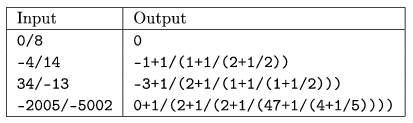Mathematical and Physical Journal
for High Schools
Issued by the MATFUND Foundation
 Already signed up? New to KöMaL?

#Problem I. 115. (November 2005)

I. 115. It is well-known that every rational number x can uniquely be represented as a finite continued fraction:where a0 is an integer, and a1,...,an are positive integers, further an>1. The terms of the continued fraction are obtained by a simple greedy algorithm. Number a0 can only be the integer part of x. If x is an integer, then we are ready. Otherwise, if x is not an integer, we have, with, and now we have to find a representation only for y.

Write a program that converts ordinary fractions into their continued fraction form.

The program should read the ordinary fractions from the standard input (i.e. from the keyboard). Every line will contain a fraction of the form a/b, where a and b are integers with at most 4 digits. Your program should display the continued fraction representation of these numbers on the standard output (i.e. on the monitor), using parentheses as in the example. Your program should stop if the standard input can not be read (end of file''), or the input is an empty line.

See the example.The source code of your program (i115.pas, i115.c, ...) should be submitted.

(10 pont)

Deadline expired on December 15, 2005.

### Statistics:

 18 students sent a solution. 10 points: Balambér Dávid, Czigler András, Kiss Dániel Miklós, Szoldatics András, Szolnoki Lénárd, Véges Márton, Vincze János. 9 points: Gyüre Balázs, Szentandrási István. 8 points: 4 students. 7 points: 1 student. 3 points: 1 student. 1 point: 1 student. 0 point: 2 students.

Problems in Information Technology of KöMaL, November 2005